# Creating equations with MathML

It is possible to add scientific equations to your drills using the MathML standard. MathML is an XML-based language for describing mathematical notation. This page is by no means a MathML manual, but simply a quick introduction to MathML and how it applies to Drillster.

## Managing equations

Equations are added to a drill by simply typing in or copying and pasting the MathML XML code into the edit screen. If Drillster recognizes your code as valid MathML, the text is automatically converted into a graphical representation of the equation. If your code contains an error, or if Drillster is unable to parse your MathML code, the code is saved as literal text instead. No error messages are shown.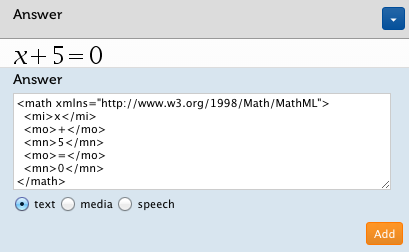Since the equation is converted to an image, it is not possible to edit the equation contents once an equation has been created. Changing an equation is best done by adding an alternative to the incorrect equation with your corrections, and subsequently deleting the incorrect alternative. For this reason, it is always advisable to keep a separate (text) file with your equation definitions.

An equation can be removed simply by clicking the small '×' next to the equation image, just like you would remove a regular image.

Equations can also be inserted as incorrect alternatives. These equations are given a red border in the editor.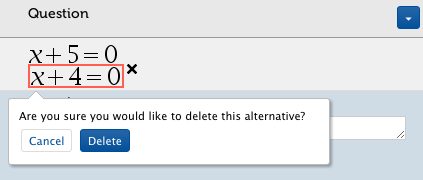It is possible to add a caption to an equation by clicking on the equation image.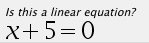Since equations are handled as images by Drillster, there is no way an equation can be entered as the answer to an open-ended question.

## Basic structure of an equation

In order for Drillster to recognize a piece of XML as MathML, all equations must have the following basic structure:

```<math xmlns="http://www.w3.org/1998/Math/MathML">
...
[/itex]```

The XML preamble and DOCTYPE should not be included. A valid equation will always start with the `math` tag.

MathML can be used to describe both the presentation and the meaning of an equation. Drillster uses the presentation variant of MathML. The `mode="display"` attribute is not required.

## Examples

The obvious use for MathML is mathematics, but MathML has something to offer for chemistry and physics as well. Here are some examples of MathML code, with their graphical representation. In order to see the full MathML code, just hover over the code snippets with your mouse.

### Mathematics

A very simple example: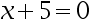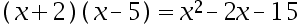This is the well known equation for solving quadratic polynomials: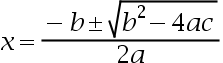Using Fractions: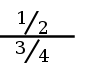`<math display='block'>  <mfrac linethickness='0.2ex'>  <mrow> <mspace width = '1em'/> <mfrac bevelled='true'>  <mn>1</mn>  <mn>2</mn>  </mfrac>  <mspace width = '1em' /> </mrow> <mrow> <mspace width = '1em' /> <mfrac bevelled='true'> <mn>3</mn> <mn>4</mn> </mfrac> <mspace width = '1em' /> </mrow> </mfrac> [/itex] `

Change "true" to "false" to change the way of using the fraction

Matrices are defined as follows: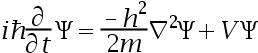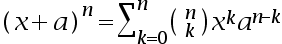It is even possible to include colors in equations: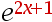### Reaction equations

MathML can also be used for reaction equations in chemistry and physics.

This equation describes the combustion of methane: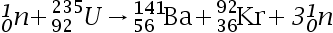An ionic reaction: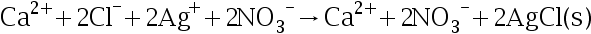Fission of uranium:## Authoring Tools

Manually typing in MathML code is not always easy. Luckily there are many external tools available that enable users to design their equations with a graphical user interface, and subsequently create the MathML code as a file export or through copying and pasting.

These products have been tested to work correctly with Drillster:

• Online MathML conversion tool by Wolfram Research allows direct conversion to MathML from Mathematica notation and standard text-based notation.
• MathType by Design Science allows you to draw an equation with a pen, digitize it and copy and paste the equation into Drillster in MathML format.
• Microsoft Word contains an equation editor for easy authoring of equations. Copy and paste your equations directly into Drillster. Make sure you select 'MathML' as the standard clipboard format for equations. The standard setting does not work.
• OpenOffice has native MathML support.

There are many more free and paid alternatives available. Please let us know if you have good experiences with another product that you feel should be listed here.